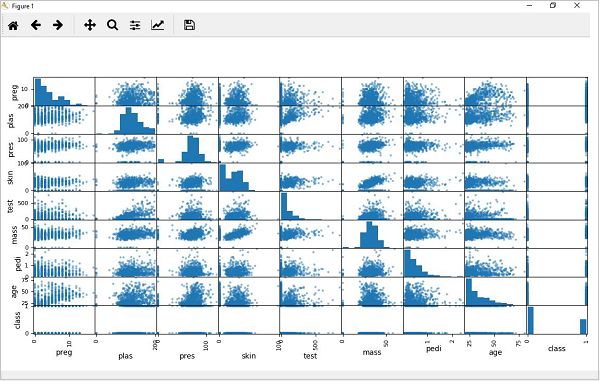# ML - Understanding Data with Visualization

## Introduction

In the previous chapter, we have discussed the importance of data for Machine Learning algorithms along with some Python recipes to understand the data with statistics. There is another way called Visualization, to understand the data.

With the help of data visualization, we can see how the data looks like and what kind of correlation is held by the attributes of data. It is the fastest way to see if the features correspond to the output. With the help of following Python recipes, we can understand ML data with statistics.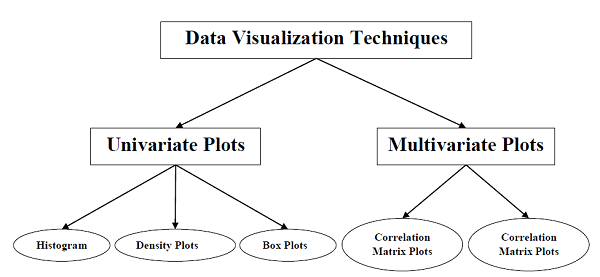## Univariate Plots: Understanding Attributes Independently

The simplest type of visualization is single-variable or “univariate” visualization. With the help of univariate visualization, we can understand each attribute of our dataset independently. The following are some techniques in Python to implement univariate visualization −

### Histograms

Histograms group the data in bins and is the fastest way to get idea about the distribution of each attribute in dataset. The following are some of the characteristics of histograms −

• It provides us a count of the number of observations in each bin created for visualization.

• From the shape of the bin, we can easily observe the distribution i.e. weather it is Gaussian, skewed or exponential.

• Histograms also help us to see possible outliers.

### Example

The code shown below is an example of Python script creating the histogram of the attributes of Pima Indian Diabetes dataset. Here, we will be using hist() function on Pandas DataFrame to generate histograms and matplotlib for ploting them.

from matplotlib import pyplot
path = r"C:\pima-indians-diabetes.csv"
names = ['preg', 'plas', 'pres', 'skin', 'test', 'mass', 'pedi', 'age', 'class']
data.hist()
pyplot.show()


## Output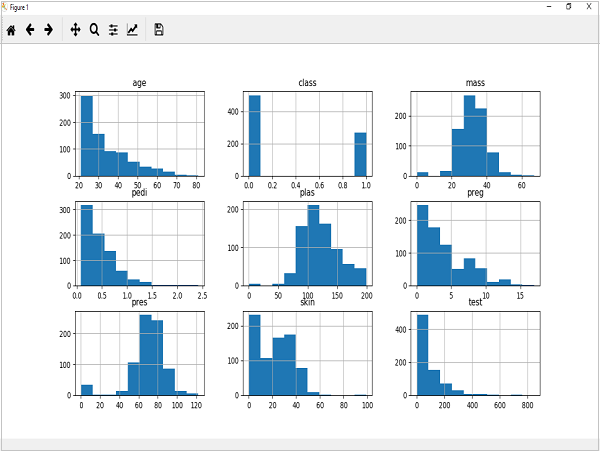The above output shows that it created the histogram for each attribute in the dataset. From this, we can observe that perhaps age, pedi and test attribute may have exponential distribution while mass and plas have Gaussian distribution.

## Density Plots

Another quick and easy technique for getting each attributes distribution is Density plots. It is also like histogram but having a smooth curve drawn through the top of each bin. We can call them as abstracted histograms.

### Example

In the following example, Python script will generate Density Plots for the distribution of attributes of Pima Indian Diabetes dataset.

from matplotlib import pyplot
path = r"C:\pima-indians-diabetes.csv"
names = ['preg', 'plas', 'pres', 'skin', 'test', 'mass', 'pedi', 'age', 'class']
data.plot(kind='density', subplots=True, layout=(3,3), sharex=False)
pyplot.show()


### Output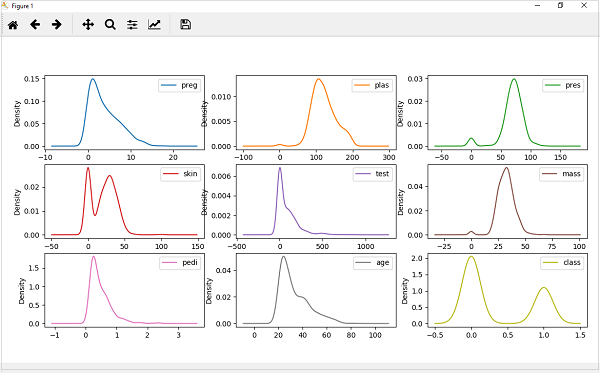From the above output, the difference between Density plots and Histograms can be easily understood.

## Box and Whisker Plots

Box and Whisker plots, also called boxplots in short, is another useful technique to review the distribution of each attribute’s distribution. The following are the characteristics of this technique −

• It is univariate in nature and summarizes the distribution of each attribute.

• It draws a line for the middle value i.e. for median.

• It draws a box around the 25% and 75%.

• It also draws whiskers which will give us an idea about the spread of the data.

• The dots outside the whiskers signifies the outlier values. Outlier values would be 1.5 times greater than the size of the spread of the middle data.

### Example

In the following example, Python script will generate Density Plots for the distribution of attributes of Pima Indian Diabetes dataset.

from matplotlib import pyplot
path = r"C:\pima-indians-diabetes.csv"
names = ['preg', 'plas', 'pres', 'skin', 'test', 'mass', 'pedi', 'age', 'class']
data.plot(kind='box', subplots=True, layout=(3,3), sharex=False,sharey=False)
pyplot.show()


### Output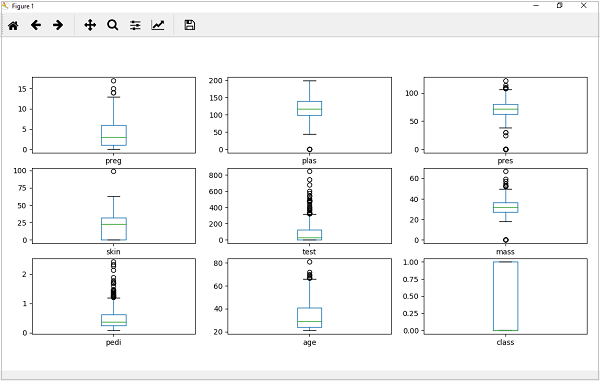From the above plot of attribute’s distribution, it can be observed that age, test and skin appear skewed towards smaller values.

## Multivariate Plots: Interaction Among Multiple Variables

Another type of visualization is multi-variable or “multivariate” visualization. With the help of multivariate visualization, we can understand interaction between multiple attributes of our dataset. The following are some techniques in Python to implement multivariate visualization −

## Correlation Matrix Plot

Correlation is an indication about the changes between two variables. In our previous chapters, we have discussed Pearson’s Correlation coefficients and the importance of Correlation too. We can plot correlation matrix to show which variable is having a high or low correlation in respect to another variable.

### Example

In the following example, Python script will generate and plot correlation matrix for the Pima Indian Diabetes dataset. It can be generated with the help of corr() function on Pandas DataFrame and plotted with the help of pyplot.

from matplotlib import pyplot
import numpy
Path = r"C:\pima-indians-diabetes.csv"
names = ['preg', 'plas', 'pres', 'skin', 'test', 'mass', 'pedi', 'age', 'class']
correlations = data.corr()
fig = pyplot.figure()
cax = ax.matshow(correlations, vmin=-1, vmax=1)
fig.colorbar(cax)
ticks = numpy.arange(0,9,1)
ax.set_xticks(ticks)
ax.set_yticks(ticks)
ax.set_xticklabels(names)
ax.set_yticklabels(names)
pyplot.show()


### Output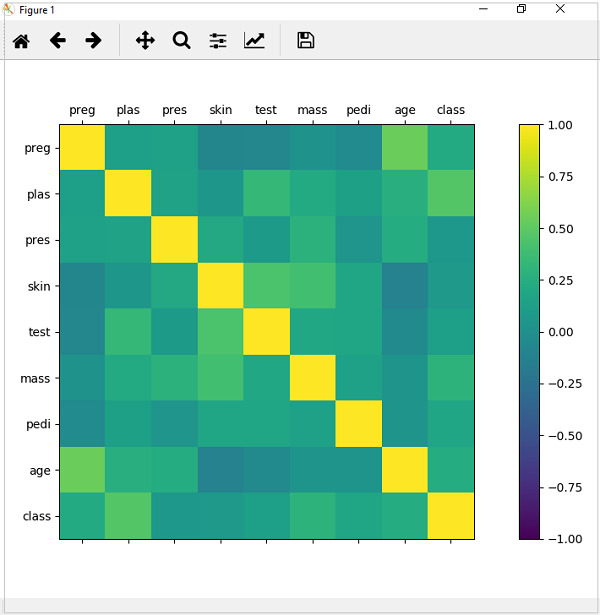From the above output of correlation matrix, we can see that it is symmetrical i.e. the bottom left is same as the top right. It is also observed that each variable is positively correlated with each other.

## Scatter Matrix Plot

Scatter plots shows how much one variable is affected by another or the relationship between them with the help of dots in two dimensions. Scatter plots are very much like line graphs in the concept that they use horizontal and vertical axes to plot data points.

### Example

In the following example, Python script will generate and plot Scatter matrix for the Pima Indian Diabetes dataset. It can be generated with the help of scatter_matrix() function on Pandas DataFrame and plotted with the help of pyplot.

from matplotlib import pyplot